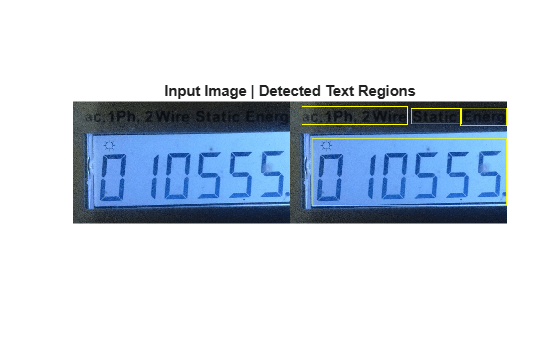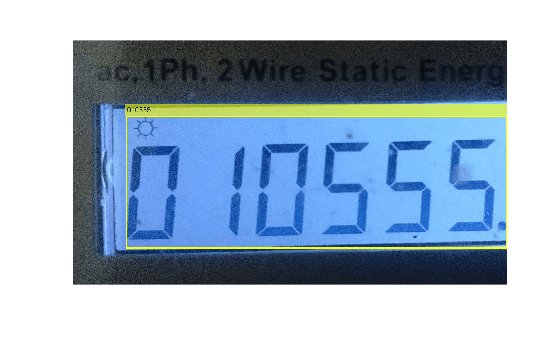# Recognize Seven-Segment Digits Using OCR

This example shows how to recognize seven-segment digits in an image by using optical character recognition (OCR). In the example, you use the `detectTextCRAFT` function and region properties to detect the seven-segment text regions in the image. Then, you use OCR to recognize the seven-segment digits in the detected text regions.

Read an image into the MATLAB® workspace.

`img = imread('meterreading.jpg');`

### Detect Seven-Segment Text Regions

Detect text regions in the input image by using the `detectTextCRAFT` function. The `CharacterThreshold` value is the region threshold to use for localizing each character in the image. The `LinkThreshold` value is the affinity threshold that defines the score for grouping two detected texts into a single instance. You can fine-tune the detection results by modifying the region and affinity threshold values. Increase the value of the affinity threshold for more word-level and character-level detections. For information about the effect of the affinity threshold on the detection results, see the Detect Characters by Modifying Affinity Threshold example.

Set the value of the affinity threshold to 0.005. The default value for the region threshold is 0.4. The output is a set of bounding boxes that localize the text regions in the input image. The bounding box specifies the spatial coordinates of the detected text regions in the image and is a vector of form [`x`, `y`, `width`, `height`]. The vector specifies the upper left corner and size of the detected region in pixels.` `

`bbox = detectTextCRAFT(img,LinkThreshold=0.005);`

Draw the output bounding boxes on the image by using the `insertShape` function.

`Iout = insertShape(img,"rectangle",bbox,LineWidth=4);`

Display the input image and the output text detections.

```figure montage({img;Iout}); title("Input Image | Detected Text Regions")```In the input image, the seven-segment text region occupies the maximum area. Use the area of the detected bounding boxes to extract the seven-segment text region.

Compute the area of the bounding boxes and find the bounding box with maximum area.

```bboxArea = bbox(:,3).*bbox(:,4); [value,indx]= max(bboxArea);```

Extract the text region with maximum bounding box area from the input image.

```roi = bbox(indx,:); extractedImg = img(roi(2):roi(2)+roi(4),roi(1):roi(1)+roi(3),:);```

Display the extracted seven-segment text region.

```figure imshow(extractedImg) title('Extracted Seven-Segment Text Region')```### Recognize Seven-Segment Digits

Recognize the seven-segment digits in the detected text region by using `ocr` function. Set the value of the `Model` name-value argument to "`seven-segment"`. The output is an `ocrText` object containing information about the recognized text, the recognition confidence, and the location of the text in the original image.

`output = ocr(img,roi,Model="seven-segment")`
```output = ocrText with properties: Text: '810000...' CharacterBoundingBoxes: [17x4 double] CharacterConfidences: [17x1 single] Words: {2x1 cell} WordBoundingBoxes: [2x4 double] WordConfidences: [2x1 single] TextLines: {2x1 cell} TextLineBoundingBoxes: [2x4 double] TextLineConfidences: [2x1 single] ```

Display the recognized seven-segment digits. You can notice that OCR detects two bounding boxes enclosing the text regions and recognizes the digits in each region.

`disp([output.Words])`
``` {'810000' } {'0110555.'} ```
```Iocr = insertObjectAnnotation(img,"Rectangle",output.WordBoundingBoxes,output.Words,LineWidth=4,FontSize=20); figure imshow(Iocr)```### Challenges Obtaining Accurate Results

The main challenges in accurate recognition of the seven-segment digits are the segmentation of text regions and the choice of the `LayoutAnalysis` name-value argument of `ocr` function.

As a preprocessing step, the `ocr` function performs binarization to segment the text regions from the background. Due to the nature of the seven-segment text images, the binarized text regions have disconnected pixels. If the distance between the pixels disconnected along the vertical direction is large and the value of the `LayoutAnalysis` parameter is set to "`block`", the `ocr` function considers the input image to have multiple lines of text. Then, the `ocr` function groups each line of text into a region and recognizes the digits within each region. As a result, the recognition results might be inaccurate. In such cases, you can improve the recognition accuracy by selecting a proper value for the `LayoutAnalysis` parameter.

### Improve Results Using `LayoutAnalysis` Parameter

If the detected image region consists of only one line of seven-segment text, you can set the `LayoutAnalysis` name-value argument to "`word`", "`character`", or "`line`" in order to obtain good recognition results. For more details about how to select the value for `LayoutAnalysis` name-value argument, see `ocr`.

The input image contains a group of seven-segment digits. To recognize all the digits in the group, set the value of the `LayoutAnalysis` name-value argument to "`word`". Compute the OCR results.

`output = ocr(img,roi,Model="seven-segment",LayoutAnalysis="word")`
```output = ocrText with properties: Text: '010555....' CharacterBoundingBoxes: [9x4 double] CharacterConfidences: [9x1 single] Words: {'010555.'} WordBoundingBoxes: [149 213 1057 365] WordConfidences: 0.4774 TextLines: {'010555.'} TextLineBoundingBoxes: [149 213 1057 365] TextLineConfidences: 0.4774 ```

Display the recognized seven-segment digits.

`disp([output.Words])`
``` {'010555.'} ```

Draw the output bounding boxes on the image by using the `insertObjectAnnotation` function. Display the recognition results. You can notice that the seven-segment text region in the image is well localized and the digits are recognized correctly.

```Iocr = insertObjectAnnotation(img,"Rectangle",output.WordBoundingBoxes,output.Words,LineWidth=4,FontSize=20); figure imshow(Iocr)```### Further Exploration

• If the detected text region consist of multiple lines of seven-segment texts, set the `LayoutAnalysis` name-value argument to "b`lock`" for optimal results.

• You can improve the recognition results by accurately localizing and segmenting the seven-segment text regions in a given image. Though you can use the `detectTextCRAFT` function for detecting the text regions, you will have to manually select the appropriate region threshold and affinity threshold values for good detection results. Alternatively, you can use the Color Thresholder or Image Segmenter apps to interactively segment the desired text regions in the image.

• If the segmented region contain outliers, use morphological operations to preprocess the image before performing OCR. For an example, see the Image Pre-processing and ROI-based Processing Techniques demonstrated in the Recognize Text Using Optical Character Recognition (OCR) example. The Improve Recognition Results section in Automatically Detect and Recognize Text Using Pretrained CRAFT Network and OCR example also demonstrates the image preprocessing techniques that you can use for improving recognition results if the image contain multiple lines of texts.